### IMO Shortlist 2008 problem C6

Kvaliteta:
Avg: 0,0
Težina:
Avg: 8,0
For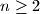$n\ge 2$, let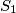$S_1$,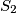$S_2$,$\ldots$,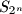$S_{2^n}$ be$2^n$ subsets of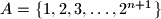$A = \{1, 2, 3, \ldots, 2^{n + 1}\}$ that satisfy the following property: There do not exist indices$a$ and$b$ with$a < b$ and elements$x$,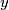$y$,$z\in A$ with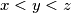$x < y < z$ and$y$,$z\in S_a$, and$x$,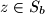$z\in S_b$. Prove that at least one of the sets$S_1$,$S_2$,$\ldots$,$S_{2^n}$ contains no more than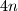$4n$ elements.

Proposed by Gerhard Woeginger, Netherlands
Izvor: Međunarodna matematička olimpijada, shortlist 2008